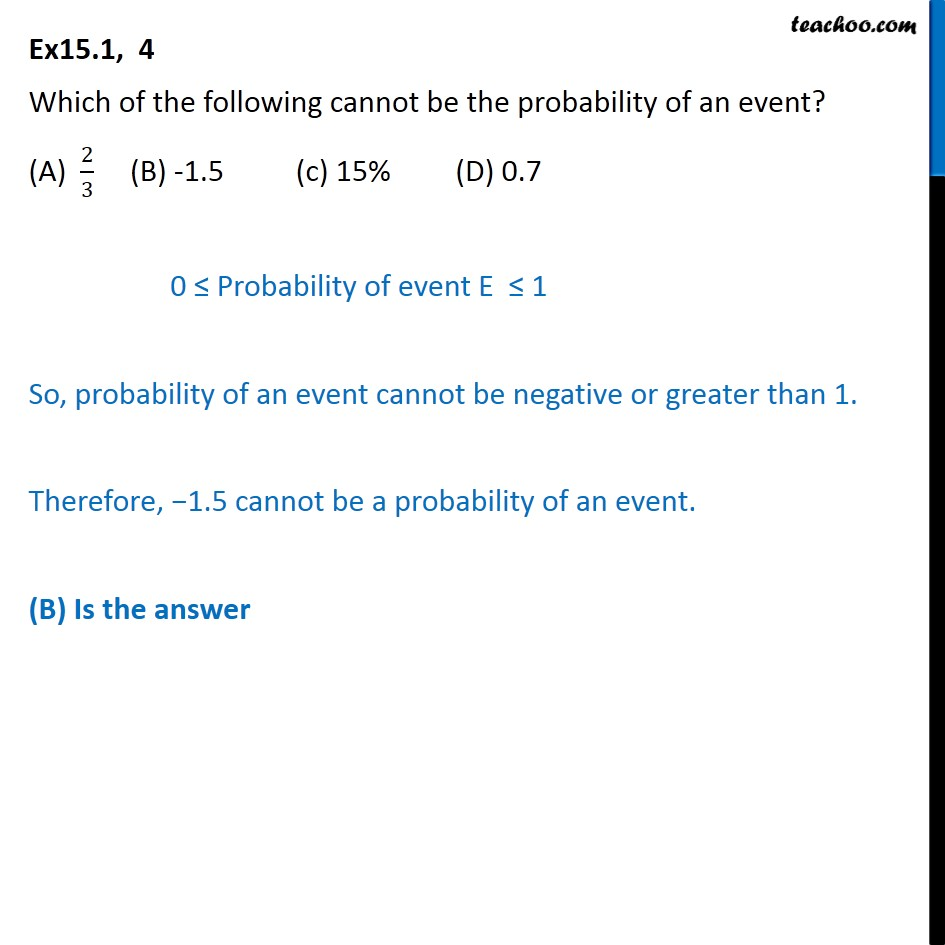1. Chapter 15 Class 10 Probability
2. Serial order wise
3. Ex 15.1

Transcript

Ex15.1, 4 Which of the following cannot be the probability of an event? (A) 2/3 (B) -1.5 (c) 15% (D) 0.7 0 ≤ Probability of event E ≤ 1 So, probability of an event cannot be negative or greater than 1. Therefore, −1.5 cannot be a probability of an event. (B) Is the answer

Ex 15.1